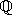## CGAL::Simple_cartesian<FieldNumberType>

#include <CGAL/Simple_cartesian.h>

### Definition

A model for a Kernel using Cartesian coordinates to represent the geometric objects. In order for Simple_cartesian<FieldNumberType> to model Euclidean geometry in E2 and/or E3, for some mathematical field E (e.g., the rationalsor the reals), the template parameter FieldNumberType must model the mathematical field E. That is, the field operations on this number type must compute the mathematically correct results. If the number type provided as a model for FieldNumberType is only an approximation of a field (such as the built-in type double), then the geometry provided by the kernel is only an approximation of Euclidean geometry.

Kernel

### Types

 typedef FieldNumberType FT; typedef FieldNumberType RT;

### Implementation

In contrast to Cartesian, no reference counting is used internally. This eases debugging, but may slow down algorithms that copy objects intensively.